# LeetCode 初级算法之链表，看看你都学会了吗？

theme: juejin highlight: xcode

# 题一：删除链表中的节点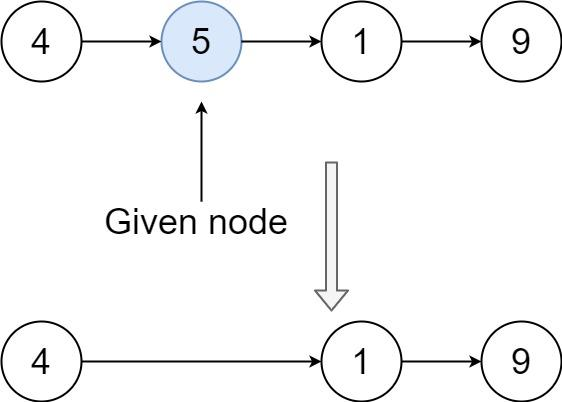```输入：head = [4,5,1,9], node = 5 输出：[4,1,9] 解释：指定链表中值为 5 的第二个节点，那么在调用了你的函数之后，该链表应变为 4 -> 1 -> 9```

## 解题思路：直接替换

```swift func deleteNode(_ node: ListNode?, _ head: ListNode?) { node?.val = node?.next?.val ?? 0 node?.next = node?.next?.next ?? nil let head = head }```

# 题二：删除链表的倒数第N个节点

## 解题思路：递归、双循环、快慢指针

### 递归

```swift func removeNthFromEnd(_ head: ListNode?, _ n: Int) -> ListNode? { let pos = length(head, n) if pos == n { return head?.next } return head }

func length(_ node: ListNode?, _ n: Int) -> Int { if node?.next == nil { return 1 } /// 获取前面一个节点 let pos = length(node?.next, n) + 1 if pos == n+1 { print("(pos)") node?.next = node?.next?.next } return pos } ```

### 双循环

```swift func removeNthFromEnd(_ head: ListNode?, _ n: Int) -> ListNode? { let pos = length(head, n) if pos == n { return head?.next } return head }

func length(_ node: ListNode?, _ n: Int) -> Int { if node?.next == nil { return 1 } /// 获取前面一个节点 let pos = length(node?.next, n) + 1 if pos == n+1 { print("(pos)") node?.next = node?.next?.next } return pos } ```

### 快慢指针

`fast``slow`两个指针，`fast`先走`n`次，然后和`slow`一起移动。 `slow``fast`相距`n`， 当`fast`先走到链表底部，`slow`就是要删除的对象；

```swift func removeNthFromEnd3(_ head: ListNode?, _ n: Int) -> ListNode? { var fast = head var slow = head var index = n /// jNode 移位 n while index > 0 { fast = fast?.next index-=1 let j = fast } if fast?.next == nil { return head?.next } /// 开始遍历 while fast?.next != nil { fast = fast?.next slow = slow?.next } slow?.next = slow?.next?.next return head }```

# 题三：反转链表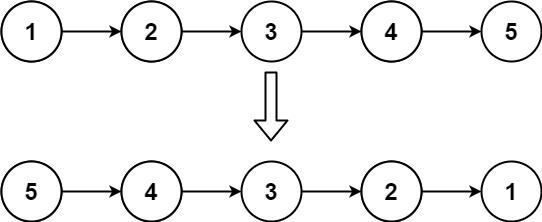```输入： head = [1,2,3,4,5] 输出： [5,4,3,2,1]```

## 解题思路：栈、递归、双指针

### 栈操作

```swift func reverseList(_ head: ListNode?) -> ListNode? { /// 全部入栈 var node = head var stack = Stack<ListNode>() while node != nil { if let node = node { stack.push(element: node) } node = node?.next } /// 第一个出栈的节点是新的表头 node = stack.pop() /// 新的表头 var newHead = node while !stack.isEmpty() { let tempNode = stack.pop() node?.next = tempNode node = tempNode } node?.next = nil return newHead }```

### 双指针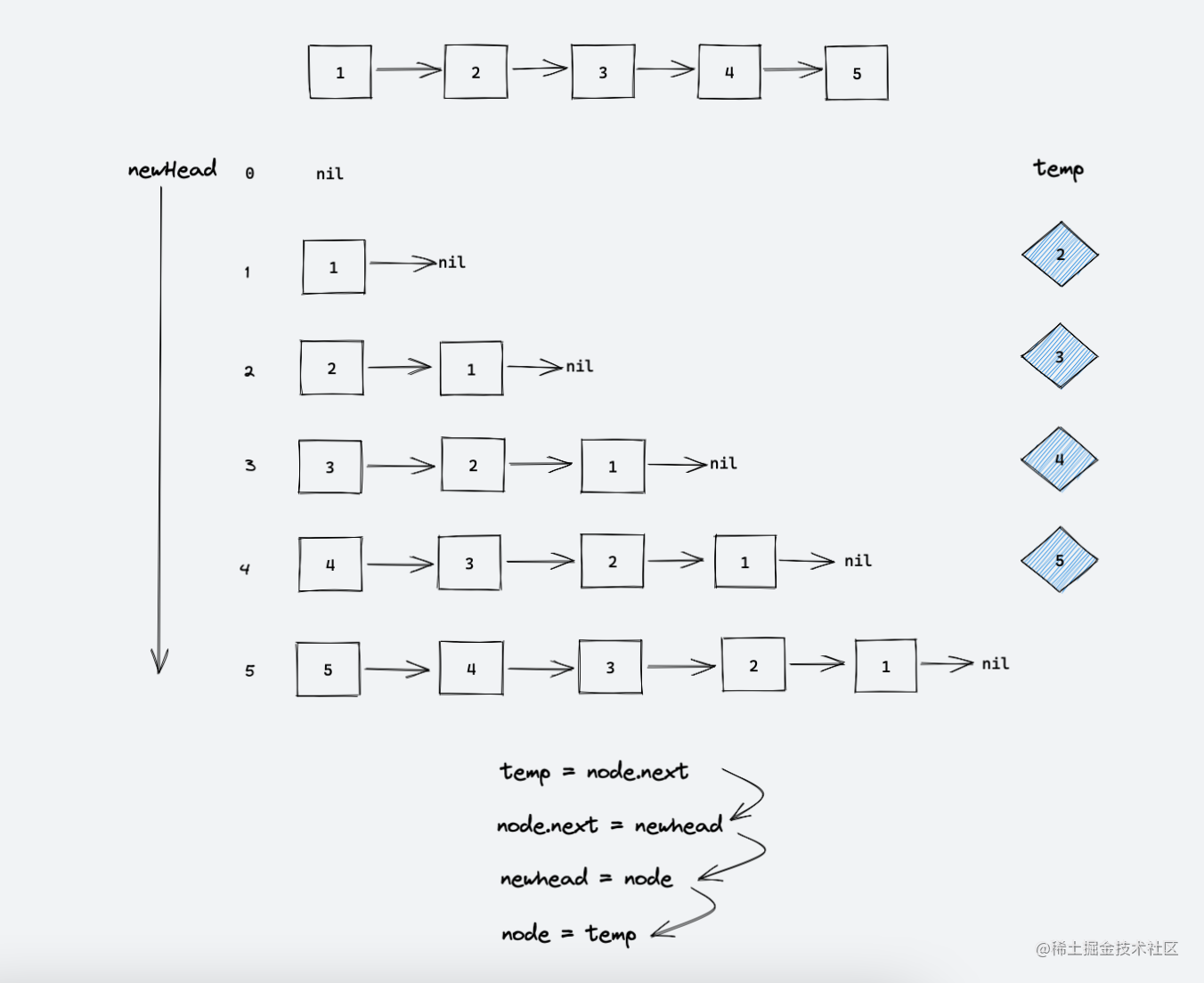```swift func reverseList(_ head: ListNode?) -> ListNode? { var node = head var newhead: ListNode? = nil while node != nil { let temp = node?.next node?.next = newhead newhead = node node = temp } return newhead }```

# 题四：合并两个有序链表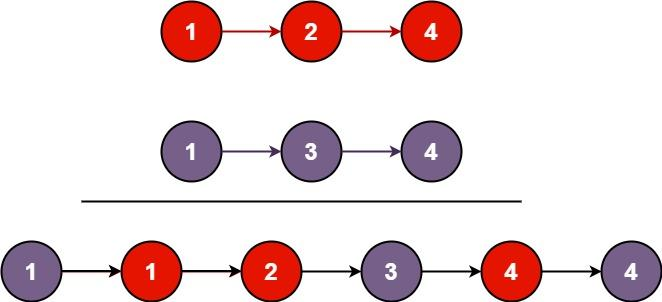```输入： l1 = [1,2,4], l2 = [1,3,4] 输出： [1,1,2,3,4,4]```

## 解题思路：双指针

```swift func mergeTwoLists(_ list1: ListNode?, _ list2: ListNode?) -> ListNode? { var node1 = list1 var node2 = list2 var newHead = ListNode(0) var cur: ListNode? = newHead while node1 != nil && node2 != nil { let val1 = node1?.val ?? 0 let val2 = node2?.val ?? 0 if val1 <= val2 { cur?.next = node1 node1 = node1?.next } else { cur?.next = node2 node2 = node2?.next } cur = cur?.next } cur?.next = node1 == nil ? node2 : node1 let head = newHead.next return head }```

# 题五：回文链表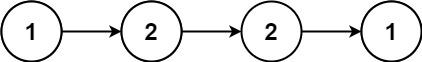```输入： head = [1,2,2,1] 输出： true```

## 解题思路：栈操作、递归、反转后半链表

### 栈操作

```swift func isPalindrome(_ head: ListNode?) -> Bool { var node = head var stack = Stack<Int>() var length = 0 while node != nil { if let node = node { stack.push(element: node.val) } node = node?.next length+=1 } node = head length/=2 while length > 0 { if node?.val != stack.pop() { return false } node = node?.next length-=1 } return true }```

### 反转后半链表

```swift func isPalindrome(_ head: ListNode?) -> Bool { var fast = head var slow = head while fast != nil, fast?.next != nil { fast = fast?.next?.next slow = slow?.next } /// 奇数就选下一个 if fast != nil { slow = slow?.next } var newNode = reverse(slow) var node = head while newNode != nil { if newNode?.val != node?.val { return false } newNode = newNode?.next node = node?.next } return true }```

# 题六：环形链表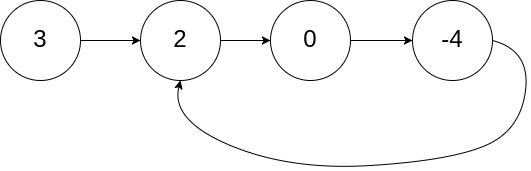```输入： head = [3,2,0,-4], pos = 1 输出： true 解释： 链表中有一个环，其尾部连接到第二个节点。``` 示例 2：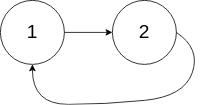```输入： head = , pos = -1 输出： false 解释： 链表中没有环。```

## 解题思路：集合法、快慢指针

### 集合法

```swift func hasCycle(_ head: ListNode?) -> Bool { var node = head?.next var set = Set<ListNode>() while node != nil { if set.contains(node!) { return true } else { set.insert(node!) } node = node?.next } return false }```